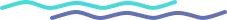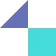# Activation Functions in Neural Networks

Activation functions are a crucial part of neural networks and play a key role in determining the output of a neuron. Activation functions are used to introduce non-linearity into the network, allowing it to model complex relationships in the data.

Activation functions are an important part of neural networks, and their selection can have a significant impact on the performance of the model. It's important to carefully consider the characteristics of the data and the specific requirements of the task when selecting an activation function.

In this blog, we'll explore some of the most common activation functions and discuss their characteristics and applications.

### Types of Activation Functions

#### Sigmoid function

The sigmoid function is a non-linear activation function that takes in any input value and squashes it between the range of 0 and 1.

It is defined as:

f(x) = 1 / (1 + e-x)

The sigmoid function has the property of being differentiable, which makes it useful for backpropagation during training.

However, it has some limitations, such as the output being saturating for large positive or negative values, which can slow down the training process.

#### Tanh function

The tanh function is another non-linear activation function that takes in any input value and squashes it between the range of -1 and 1.

It is defined as:

f(x) = 2 / (1 + e-2x) - 1

The tanh function has the advantage of being zero-centered, which can make the learning process more efficient.

However, it also has the drawback of saturating for large positive or negative values, similar to the sigmoid function.

#### ReLU function

The ReLU (Rectified Linear Unit) function is a non-linear activation function that takes in any input value and outputs the value if it is positive, or 0 if it is negative.

It is defined as:

f(x) = max(0, x)

The ReLU function is simple to implement and has the advantage of not saturating for large input values.

However, it can suffer from the problem of "dying ReLUs," where neurons with negative input values stop learning and become inactive.

#### Leaky ReLU function

The Leaky ReLU function is a variant of the ReLU function that introduces a small positive slope for negative input values, rather than outputting 0.

It is defined as:

f(x) = max(0.01x, x)

The Leaky ReLU function addresses the problem of dying ReLUs by allowing neurons with negative input values to still learn, albeit at a slower rate.

#### Softmax function

The Softmax function is a non-linear activation function commonly used in classification tasks. It takes in a vector of real values and converts it into a probability distribution, where the sum of all values is 1.

It is defined as:

f(x) = ex / sum(ex)

The Softmax function is useful for converting the output of a neural network into a probability distribution over multiple classes, allowing the network to make predictions by selecting the class with the highest probability.

#### Swish function

The Swish function is a non-linear activation function defined as:

f(x) = x * sigmoid(x)

It was introduced as an alternative to the ReLU function and has been shown to improve the performance of neural networks in some tasks.

The Swish function has the advantage of being self-gated, meaning that it can learn to adaptively adjust its output based on the input data.

#### ELU (Exponential Linear Unit) function

The ELU function is a non-linear activation function defined as:

f(x) = x if x > 0, otherwise alpha * (ex - 1)

where alpha is a hyperparameter that controls the slope of the function for negative input values.

The ELU function has the advantage of not having the problem of "dying ReLUs," where neurons with negative input values stop learning and become inactive. It has been shown to improve the performance of neural networks in some tasks.

#### GELU (Gaussian Error Linear Unit) function

The GELU function is a non-linear activation function defined as:

f(x) = x * sigmoid((2/π) * x)

It was introduced as an alternative to the ReLU function and has been shown to improve the performance of neural networks in some tasks.

The GELU function has the advantage of being self-normalizing, meaning that it can learn to adjust its output distribution to match the input data distribution.

#### Maxout function

The Maxout function is a non-linear activation function defined as:

f(x) = max(x1, x2, ..., xn)

where x1, x2, ..., xn are the input values.

The Maxout function has the advantage of being able to learn which input value to select, allowing it to adaptively adjust its output based on the input data. It has been shown to improve the performance of neural networks in some tasks.

#### PReLU (Parametric ReLU) function

The PReLU function is a variant of the ReLU function that introduces a learnable parameter alpha, which controls the slope of the function for negative input values.

It is defined as:

f(x) = x if x > 0, otherwise alpha * x

The PReLU function addresses the problem of dying ReLUs by allowing neurons with negative input values to still learn, albeit at a slower rate.

The parameter alpha can be learned during training, allowing the PReLU function to adaptively adjust its output based on the input data.

It's worth noting that this is not an exhaustive list and there are other activation functions that are less commonly used. The choice of activation function can depend on the specific requirements of the task and the characteristics of the data.

a. Threshold function
b. Logistic function
c. Arctan function
d. Softplus function
e. Softsign function
f. ISRU (Inverse Square Root Unit) function
g. SELU (Scaled Exponential Linear Unit) function
h. SReLU (Smooth ReLU) function
i. Hard sigmoid function
j. Bent identity function

Are you ready to take your business to the next level with the power of AI? Look no further than Tooliqa!
Our team of experts is dedicated to helping businesses like yours simplify and automate their processes through the use of AI, computer vision, deep learning, and top-notch product design UX/UI.

We have the knowledge and experience to guide you in using these cutting-edge technologies to drive process improvement and increase efficiency.

Let us help you unlock the full potential of AI – reach out to us at business@tooli.qa and take the first step towards a brighter future for your company.

## FAQs

Quick queries for this insight

No items found.

### Connect with our experts today for a free consultation.

Oops! Something went wrong while submitting the form.

## Subscribe to Tooliqa

Oops! Something went wrong while submitting the form.## Built for Innovators

DICEWith advanced-tech offerings designed to handle challenges at scale, Tooliqa delivers solid infrastructure and solutioning which are built for to meet most difficult enterprise-level needs.​